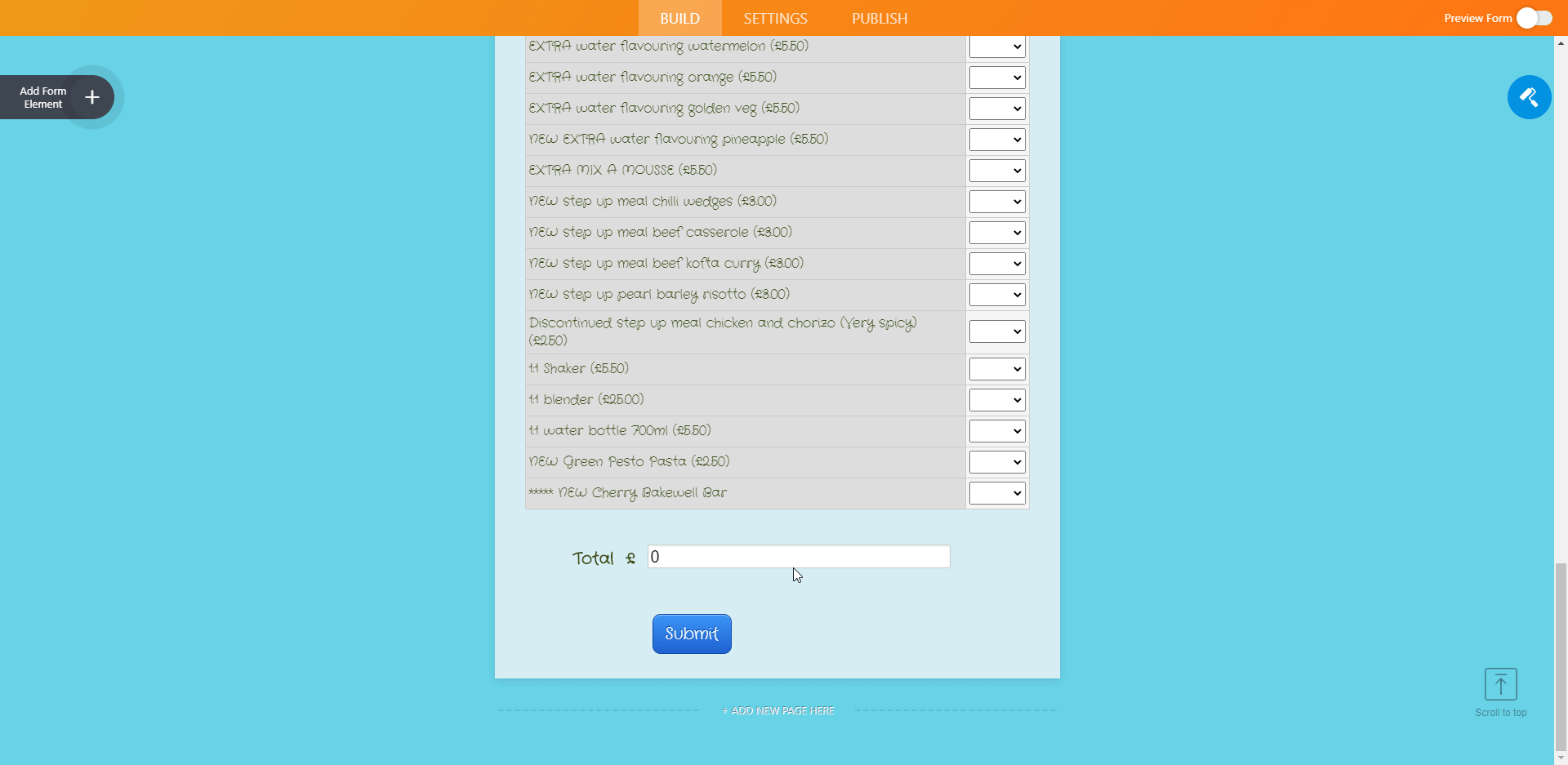# How to Delete Background Calculation in a Form

•julia.tait
Asked on June 12, 2020 at 11:48 AM

Hi,

The form I have built is for customers to order food items. There is a dropdown field for the number of items to be selected.

I only want certain fields on the form to calculate a monetary value for the items being ordered. Other fields to not add a monetary value to the overall total.

The form I am using is a modified form I have copied. All fields have a background calculation and I do not know how to delete the calculation formula in that field.

Many Thanks

Julia

•Niko_N
Answered on June 12, 2020 at 01:07 PM

Greetings Julia,

All the calculations in your form are performed by Form Calculation widget:So, for Total field it calculates and displays the following: fields with the same price are grouped, selected quantities are summed up from dropdown options, then multiplied by corresponding price and total sum is calculated.

You can update/modify that calculations according your needs. You can also check this guide:

https://www.jotform.com/help/259-How-to-Perform-Form-Calculation-Using-a-Widget

Alternatively, you can share with us exact logic/calculations you want to perform (i.e. what to get in the Total field), so we will suggest corresponding modifications.

Please check and let us know if you need any further assistance.

Thank you!